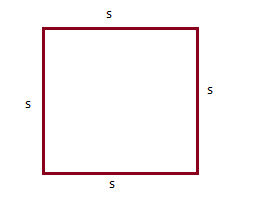Courses
Courses for Kids
Free study material
Free LIVE classes
More# A square board has an area of 121 sq.cm. What is its perimeter?

Last updated date: 20th Mar 2023
Total views: 305.4k
Views today: 3.84kVerified
305.4k+ views
Hint: Perimeter any region depends on the shape (2D) of the object surface. Perimeter of a surface is simply the measure of the length around the border of that surface. It is given that the area of a square board = 121 sq.cm.

We knew that, in a square all sides have equal length. If a side measure of the square is ‘$s$’ units, then the area of that square will be ‘ ${s^2}$ ’ sq. unitsThe perimeter of the square is equal to sum of length of all the four sides = s + s + s+ s = 4s
In the given problem, the square area is 121 sq.cm
$\Rightarrow {s^2} = 121sq.cm$
Taking square root on both sides of the above equation,
$\Rightarrow s = \sqrt {121}$
$\Rightarrow s = 11cm$
We got the side length of the given square as s=11cm.
Then perimeter is $4s = 4 \times 11cm = 44cm$
$\therefore$ The perimeter of the square with area 121 sq. units = 44cm

Note: We first need to find the lengths of all sides of the given shape to find the perimeter. In the problem square shape is given, so all side measures will be equal. We found a side measure from the area of the square using the basic logic that, in any square area is equal to (side $\times$ side).